## Java Tutorial - Hangman[Intermediate]

Logged in as: GuestAll Forums >> [Gaming Community] >> [Legends and Lore] >> Artists of Legend >> Art Academy >> Java Tutorial - Hangman[Intermediate]
Forum LoginMessage << Older Topic   Newer Topic >>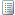2/11/2010 19:11:08
Sephiroth12
MemberWell... It's been a while since I've posted anything, so I thought I'd start again :) Anyway, this is a project I did completely from scratch, and it took me a few hours(on and off; I have a life lol). But yeah I'd recommend that you have a basic knowledge of how Java works or at least any other OOP language by those means, but if not, don't stress; now's as good a time as any to learn.

Anyway, I'm doing the rest of this in the regular black colored font due to easier legibility. But first off, requirements(recommendations, actually) for this tutorial:

• You should know a little bit about programming/coding in general
• You need to be good with math/ have a bit of logic*
• You need to have Java JDK installed. You can find it here: JDK 6 Update 18
With Java EE

• You need a Java Compiler
• THIS IS A COMMAND LINE PROGRAM; IT RUNS USING CMD

* All programs are made of logic. Well, code too, but that's not the point :P Pretty much, the easy part is learning the language; the hard part is using it. Once you've learned the language you can do endless things -- whether it's making a game, a web applet, an application, etc. But basically all you need is the logic of how it will work; what do you want it to do? What should it do if this happens? What if that happens? etc. NO PROGRAM WILL AUTOMATICALLY KNOW WHAT TO DO; YOU have to TELL it what to do; this is where the programming comes in. So that's why you need logic; your program won't do anything on it's own; everything is predetermined. This is why you should plan out everything you program before doing it... Sorry if this doesn't make sense; I'll go over this more later :|

Part 1: The Design
What is this program going to do? : This program is going to be a game called hangman; you guess the letters to a word.
So, that's the idea; let's make hangman. But where to start? First of all, you need to plan how everything will happen. I've done so with a sort of web:
Main Class(Hangman.java):
start
--Play with this difficulty
---Start the Game clas(Play.java)
Play.java:
Get the difficulty
-Set the words
--let the user guess
---If right, add the letter to the 'right' letters
---If wrong, add to the 'wrong' letters
--Lose/win
---Restart/Close
Since we need to start somewhere, we'll make a class called hangman.java:
```/*
Hangman.java
Here is the main class; where all events will be processed
*/
class Hangman
{
//This is where everything will start
}
```

So, this is the base for our Hangman game; Hangman.java. In this file, we will start the game. In this hangman there is three difficulties; Easy, Medium, and Hard. Based on the difficulty, a list of words will be chosen and the game will begin.

So, this is the beginning. Now, let's display some text:
```/*
Hangman.java
Here is the main class; where all events will be processed
*/
class Hangman
{
//This is where everything will start
public static void main(String[] args)
{
System.out.println("Hello, and welcome to Hangman!");
}
}
```

Now, this displays: Hello, and welcome to Hangman! on the screen. Next we want to create a Scanner* to take in all the text the user types:
```/*
Hangman.java
Here is the main class; where all events will be processed
*/
import java.util.Scanner;
class Hangman
{
//This is where everything will start
public static void main(String[] args)
{
Scanner input = new Scanner(System.in);
System.out.println("Hello, and welcome to Hangman!");
}
}
```

*A scanner is an object that takes the input from the keyboard and can be used to set the input to a variable.
Now whatever the user types can be used as information when we would like to use it. Next let's make it so that the user can choose the difficulty using this scanner:
```/*
Hangman.java
Here is the main class; where all events will be processed
*/
import java.util.Scanner;
class Hangman
{
//This is where everything will start
public static void main(String[] args)
{
Scanner input = new Scanner(System.in);
System.out.println("Hello, and welcome to Hangman!");
int diff = guess.nextInt();
if(diff==1)
{
Play obj = new Play();
obj.Start(diff);
}else{
if(diff==2)
{
Play obj = new Play();
obj.Start(diff);
}else{
Play obj = new Play();
obj.Start(3);
}
}
}
}
```

We just did a lot; what we did was ask the user to input the difficulty using 1-3, and based off of this it would create a Play object called obj and tell it to 'Start' using the number in which they inputted. This can also be done without the branch of if statements, but I won't use that. So, this is the Hangman.java file; not too much :) Now for the Play.java file(This is where it gets complicated...):
```//Let's start by creating the class:
public class Play
{
public Play()
{
//Don't put anything here :)
}
}
```

Now let's create the 'Start' method that we called earlier in Hangman.java:
```//Let's start by creating the class:
public class Play
{
public Play()
{
//Don't put anything here :)
}
public void Start(int diff)
{
//Do whatever with the integer diff(difficulty) here
}
}
```

Now let's starting using the difficulty:
```//Let's start by creating the class:
public class Play
{
public Play()
{
//Don't put anything here :)
}
public void Start(int diff)
{
//Do whatever with the integer diff(difficulty) here
String words[] = new String;
String hints[] = new String;
switch(diff)
{
case 1:

words = "cat";
hints = "Animal";
words = "dog";
hints = "Animal";
words = "book";
words = "breakfeast";
hints = "Meals";
words = "telephone";
hints = "Communication";
words = "mixture";
hints = "Noun";
words = "music";
hints = "Form of Expression";
words = "animal";
hints = "Think cat, dog, tiger, etc.";
words = "school";
hints = "Building";
words = "plant";
hints = "Think grass, tree, flower, etc.";
words = "pen";
hints = "Office tool.";
words = "pencil";
hints = "Office tool.";
words = "paper";
hints = "Office tool.";
words = "note";
hints = "You can pass it around.";
words = "fog";
hints = "Form of percipitation.";
words = "smoke";
hints = "Comes from fire.";
words = "bake";
hints = "Cooking.";
words = "alone";
hints = "Without Others.";
words = "drive";
hints = "Car.";
words = "town";
hints = "Form of community.";
words = "city";
hints = "Form of community.";
words = "sunny";
hints = "Sunlight.";
words = "shine";
hints = "Glisten.";
words = "polish";
hints = "Clean.";
words = "cap";
words = "hat";
break;
case 2:
words = "president";
words = "exclamation";
hints = "Shout out.";
words = "statement";
hints = "To say.";
words = "television";
hints = "You watch it.";
words = "physics";
hints = "Form of Science.";
words = "algebra";
hints = "Form of math.";
words = "geometry";
hints = "Form of math.";
words = "difficult";
hints = "Hard.";
words = "extreme";
hints = "Intense.";
words = "procedure";
hints = "Steps.";
words = "ship";
hints = "Big Boat.";
words = "soldier";
hints = "Army.";
words = "lunch";
hints = "Meal.";
words = "hockey";
hints = "Sports.";
words = "tennis";
hints = "Sports.";
words = "soccer";
hints = "Sports.";
words = "football";
hints = "Sports.";
hints = "Sports.";
words = "bias";
hints = "One sided.";
words = "magazine";
hints = "Form of book.";
words = "computer";
hints = "Microsoft.";
words = "internet";
hints = "World Wide Web.";
words = "allegedly";
hints = "Supposedly.";
words = "system";
hints = "Network.";
words = "unison";
hints = "As one.";
words = "excited";
hints = "Upbeat.";
break;
case 3:
words = "amalgamation";
hints = "Mixture.";
words = "proclomation";
hints = "Proclaim.";
words = "establishment";
hints = "Institution.";
words = "rehabilitation";
hints = "Reform.";
words = "rhinoceros";
hints = "Animal.";
words = "velociraptor";
hints = "Dinosaur.";
words = "declaration";
hints = "Declare.";
words = "announcement";
hints = "Announce.";
words = "binomial";
hints = "Form of monomial.";
words = "polynomial";
hints = "Form of trinomial.";
words = "congregation";
hints = "Group.";
words = "obligation";
hints = "Required.";
words = "structure";
hints = "Anatomy.";
words = "description";
hints = "Describe.";
words = "perscription";
hints = "Perscribe.";
words = "subscribe";
hints = "Join.";
hints = "Place.";
words = "township";
hints = "Multiple Schools.";
words = "mischievous";
hints = "Sneaky.";
words = "bewildered";
hints = "Puzzled, Confused.";
words = "accusation";
hints = "To Conclude.";
words = "designation";
hints = "Assign.";
words = "disgusting";
hints = "Nasty, Gross.";
words = "prolonged";
hints = "Extend.";
words = "restoration";
hints = "Rebuild.";
words = "regeneration";
hints = "To Be Reborn.";
}
}
}
```

Now, this may SEEM like a lot, but it's not;
What we did was create two lists; the hints, and the words(these are both arrays). Based on the difficulty, there was a switch statement, what to do if the difficulty was 1, 2, or 3. Then it set the words and hints for each difficulty in each list.

Now what we want to do is select a word from the list and start the game with this word. To do this, we need to use java.util.Random, create a guess string*, set an integer tt(timesTried)**, and create a boolean canPlay(I don't use this in the final outcome :\):
```//Let's start by creating the class:
public class Play
{
public Play()
{
//Don't put anything here :)
}
public void Start(int diff)
{
//Do whatever with the integer diff(difficulty) here
String words[] = new String;
String hints[] = new String;
switch(diff)
{
case 1:

words = "cat";
hints = "Animal";
words = "dog";
hints = "Animal";
words = "book";
words = "breakfeast";
hints = "Meals";
words = "telephone";
hints = "Communication";
words = "mixture";
hints = "Noun";
words = "music";
hints = "Form of Expression";
words = "animal";
hints = "Think cat, dog, tiger, etc.";
words = "school";
hints = "Building";
words = "plant";
hints = "Think grass, tree, flower, etc.";
words = "pen";
hints = "Office tool.";
words = "pencil";
hints = "Office tool.";
words = "paper";
hints = "Office tool.";
words = "note";
hints = "You can pass it around.";
words = "fog";
hints = "Form of percipitation.";
words = "smoke";
hints = "Comes from fire.";
words = "bake";
hints = "Cooking.";
words = "alone";
hints = "Without Others.";
words = "drive";
hints = "Car.";
words = "town";
hints = "Form of community.";
words = "city";
hints = "Form of community.";
words = "sunny";
hints = "Sunlight.";
words = "shine";
hints = "Glisten.";
words = "polish";
hints = "Clean.";
words = "cap";
words = "hat";
break;
case 2:
words = "president";
words = "exclamation";
hints = "Shout out.";
words = "statement";
hints = "To say.";
words = "television";
hints = "You watch it.";
words = "physics";
hints = "Form of Science.";
words = "algebra";
hints = "Form of math.";
words = "geometry";
hints = "Form of math.";
words = "difficult";
hints = "Hard.";
words = "extreme";
hints = "Intense.";
words = "procedure";
hints = "Steps.";
words = "ship";
hints = "Big Boat.";
words = "soldier";
hints = "Army.";
words = "lunch";
hints = "Meal.";
words = "hockey";
hints = "Sports.";
words = "tennis";
hints = "Sports.";
words = "soccer";
hints = "Sports.";
words = "football";
hints = "Sports.";
hints = "Sports.";
words = "bias";
hints = "One sided.";
words = "magazine";
hints = "Form of book.";
words = "computer";
hints = "Microsoft.";
words = "internet";
hints = "World Wide Web.";
words = "allegedly";
hints = "Supposedly.";
words = "system";
hints = "Network.";
words = "unison";
hints = "As one.";
words = "excited";
hints = "Upbeat.";
break;
case 3:
words = "amalgamation";
hints = "Mixture.";
words = "proclomation";
hints = "Proclaim.";
words = "establishment";
hints = "Institution.";
words = "rehabilitation";
hints = "Reform.";
words = "rhinoceros";
hints = "Animal.";
words = "velociraptor";
hints = "Dinosaur.";
words = "declaration";
hints = "Declare.";
words = "announcement";
hints = "Announce.";
words = "binomial";
hints = "Form of monomial.";
words = "polynomial";
hints = "Form of trinomial.";
words = "congregation";
hints = "Group.";
words = "obligation";
hints = "Required.";
words = "structure";
hints = "Anatomy.";
words = "description";
hints = "Describe.";
words = "perscription";
hints = "Perscribe.";
words = "subscribe";
hints = "Join.";
hints = "Place.";
words = "township";
hints = "Multiple Schools.";
words = "mischievous";
hints = "Sneaky.";
words = "bewildered";
hints = "Puzzled, Confused.";
words = "accusation";
hints = "To Conclude.";
words = "designation";
hints = "Assign.";
words = "disgusting";
hints = "Nasty, Gross.";
words = "prolonged";
hints = "Extend.";
words = "restoration";
hints = "Rebuild.";
words = "regeneration";
hints = "To Be Reborn.";
}
String word;
Random rand = new Random();
int w1 = rand.nextInt(26);
word = words[w1];
String hint = hints[w1];
String guess = "";
int wl = word.length()+1;
for(int i=1;i<wl;i++)
{
guess += "-";
}
//Start the guessing!
String lu = "";
int tt = 0;
boolean canPlay = true;
}
}
```

What we just did was select the word to use, and based on the amount of characters in this, a guess string is created which will have the dashes for the letters the user needs to guess. A string lu(lettersUsed) is created to hold the letters the user has already guessed. Now, let's put these into action:
```//Let's start by creating the class:
public class Play
{
public Play()
{
//Don't put anything here :)
}
public void Start(int diff)
{
//Do whatever with the integer diff(difficulty) here
String words[] = new String;
String hints[] = new String;
switch(diff)
{
case 1:

words = "cat";
hints = "Animal";
words = "dog";
hints = "Animal";
words = "book";
words = "breakfeast";
hints = "Meals";
words = "telephone";
hints = "Communication";
words = "mixture";
hints = "Noun";
words = "music";
hints = "Form of Expression";
words = "animal";
hints = "Think cat, dog, tiger, etc.";
words = "school";
hints = "Building";
words = "plant";
hints = "Think grass, tree, flower, etc.";
words = "pen";
hints = "Office tool.";
words = "pencil";
hints = "Office tool.";
words = "paper";
hints = "Office tool.";
words = "note";
hints = "You can pass it around.";
words = "fog";
hints = "Form of percipitation.";
words = "smoke";
hints = "Comes from fire.";
words = "bake";
hints = "Cooking.";
words = "alone";
hints = "Without Others.";
words = "drive";
hints = "Car.";
words = "town";
hints = "Form of community.";
words = "city";
hints = "Form of community.";
words = "sunny";
hints = "Sunlight.";
words = "shine";
hints = "Glisten.";
words = "polish";
hints = "Clean.";
words = "cap";
words = "hat";
break;
case 2:
words = "president";
words = "exclamation";
hints = "Shout out.";
words = "statement";
hints = "To say.";
words = "television";
hints = "You watch it.";
words = "physics";
hints = "Form of Science.";
words = "algebra";
hints = "Form of math.";
words = "geometry";
hints = "Form of math.";
words = "difficult";
hints = "Hard.";
words = "extreme";
hints = "Intense.";
words = "procedure";
hints = "Steps.";
words = "ship";
hints = "Big Boat.";
words = "soldier";
hints = "Army.";
words = "lunch";
hints = "Meal.";
words = "hockey";
hints = "Sports.";
words = "tennis";
hints = "Sports.";
words = "soccer";
hints = "Sports.";
words = "football";
hints = "Sports.";
hints = "Sports.";
words = "bias";
hints = "One sided.";
words = "magazine";
hints = "Form of book.";
words = "computer";
hints = "Microsoft.";
words = "internet";
hints = "World Wide Web.";
words = "allegedly";
hints = "Supposedly.";
words = "system";
hints = "Network.";
words = "unison";
hints = "As one.";
words = "excited";
hints = "Upbeat.";
break;
case 3:
words = "amalgamation";
hints = "Mixture.";
words = "proclomation";
hints = "Proclaim.";
words = "establishment";
hints = "Institution.";
words = "rehabilitation";
hints = "Reform.";
words = "rhinoceros";
hints = "Animal.";
words = "velociraptor";
hints = "Dinosaur.";
words = "declaration";
hints = "Declare.";
words = "announcement";
hints = "Announce.";
words = "binomial";
hints = "Form of monomial.";
words = "polynomial";
hints = "Form of trinomial.";
words = "congregation";
hints = "Group.";
words = "obligation";
hints = "Required.";
words = "structure";
hints = "Anatomy.";
words = "description";
hints = "Describe.";
words = "perscription";
hints = "Perscribe.";
words = "subscribe";
hints = "Join.";
hints = "Place.";
words = "township";
hints = "Multiple Schools.";
words = "mischievous";
hints = "Sneaky.";
words = "bewildered";
hints = "Puzzled, Confused.";
words = "accusation";
hints = "To Conclude.";
words = "designation";
hints = "Assign.";
words = "disgusting";
hints = "Nasty, Gross.";
words = "prolonged";
hints = "Extend.";
words = "restoration";
hints = "Rebuild.";
words = "regeneration";
hints = "To Be Reborn.";
}
String word;
Random rand = new Random();
int w1 = rand.nextInt(26);
word = words[w1];
String hint = hints[w1];
String guess = "";
int wl = word.length()+1;
for(int i=1;i<wl;i++)
{
guess += "-";
}
//Start the guessing!
String lu = "";
int tt = 0;
boolean canPlay = true;
Main(guess, tt, canPlay, lu, word, hint);
}
public void Main(String guess, int tt, boolean canPlay, String lu, String word, String hint)
{
if(tt==6)
{
canPlay = false;
Lose();
}else{
/*
Put man here
--
o |
/|\|
/ \|
_____
*/
String man[] = new String;
man = " --\n   |\n   |\n   |\n_____\n";
man = " --\n o |\n   |\n   |\n_____\n";
man = " --\n o |\n/  |\n   |\n_____\n";
man = " --\n o |\n/| |\n   |\n_____\n";
man = " --\n o |\n/|\\|\n   |\n_____\n";
man = " --\n o |\n/|\\|\n/  |\n_____\n";
man = " --\n o |\n/|\\|\n/ \\|\n_____\n";
char g1[] = guess.toCharArray();
char w2[] = word.toCharArray();
System.out.println(man);
for(int x=0;x<g1.length;x++)
{
System.out.print(g1[x]);
}
System.out.println();
Guess(guess, tt, canPlay, lu, word, g1, w2, man, hint);
}
}
}
```

We just created a method called 'Main', which will display the man on the gallows as the user progresses in the game. What happens here is it checks if the user has guessed 6 wrong letters -- and if so they lose --, shows the man on the gallows, creates two character arrays to convert the word string and guess string to character arrays so that we can tell if the word is completely done(if the guess string is equal to the word and contains no '-'s). Then we display the guess string with a for loop which loops through each character in the guess string, making it show all of the characters in the guess string. So if the guess string was "cat", then the guess character array would be "c", "a", "t", and it displays each character. Next let's create the Guess method so the user can actually guess:
```//Let's start by creating the class:
import java.util.Scanner;
import java.util.Random;
public class Play
{
public Play()
{
//Don't put anything here :)
}
public void Start(int diff)
{
//Do whatever with the integer diff(difficulty) here
String words[] = new String;
String hints[] = new String;
switch(diff)
{
case 1:

words = "cat";
hints = "Animal";
words = "dog";
hints = "Animal";
words = "book";
words = "breakfeast";
hints = "Meals";
words = "telephone";
hints = "Communication";
words = "mixture";
hints = "Noun";
words = "music";
hints = "Form of Expression";
words = "animal";
hints = "Think cat, dog, tiger, etc.";
words = "school";
hints = "Building";
words = "plant";
hints = "Think grass, tree, flower, etc.";
words = "pen";
hints = "Office tool.";
words = "pencil";
hints = "Office tool.";
words = "paper";
hints = "Office tool.";
words = "note";
hints = "You can pass it around.";
words = "fog";
hints = "Form of percipitation.";
words = "smoke";
hints = "Comes from fire.";
words = "bake";
hints = "Cooking.";
words = "alone";
hints = "Without Others.";
words = "drive";
hints = "Car.";
words = "town";
hints = "Form of community.";
words = "city";
hints = "Form of community.";
words = "sunny";
hints = "Sunlight.";
words = "shine";
hints = "Glisten.";
words = "polish";
hints = "Clean.";
words = "cap";
words = "hat";
break;
case 2:
words = "president";
words = "exclamation";
hints = "Shout out.";
words = "statement";
hints = "To say.";
words = "television";
hints = "You watch it.";
words = "physics";
hints = "Form of Science.";
words = "algebra";
hints = "Form of math.";
words = "geometry";
hints = "Form of math.";
words = "difficult";
hints = "Hard.";
words = "extreme";
hints = "Intense.";
words = "procedure";
hints = "Steps.";
words = "ship";
hints = "Big Boat.";
words = "soldier";
hints = "Army.";
words = "lunch";
hints = "Meal.";
words = "hockey";
hints = "Sports.";
words = "tennis";
hints = "Sports.";
words = "soccer";
hints = "Sports.";
words = "football";
hints = "Sports.";
hints = "Sports.";
words = "bias";
hints = "One sided.";
words = "magazine";
hints = "Form of book.";
words = "computer";
hints = "Microsoft.";
words = "internet";
hints = "World Wide Web.";
words = "allegedly";
hints = "Supposedly.";
words = "system";
hints = "Network.";
words = "unison";
hints = "As one.";
words = "excited";
hints = "Upbeat.";
break;
case 3:
words = "amalgamation";
hints = "Mixture.";
words = "proclomation";
hints = "Proclaim.";
words = "establishment";
hints = "Institution.";
words = "rehabilitation";
hints = "Reform.";
words = "rhinoceros";
hints = "Animal.";
words = "velociraptor";
hints = "Dinosaur.";
words = "declaration";
hints = "Declare.";
words = "announcement";
hints = "Announce.";
words = "binomial";
hints = "Form of monomial.";
words = "polynomial";
hints = "Form of trinomial.";
words = "congregation";
hints = "Group.";
words = "obligation";
hints = "Required.";
words = "structure";
hints = "Anatomy.";
words = "description";
hints = "Describe.";
words = "perscription";
hints = "Perscribe.";
words = "subscribe";
hints = "Join.";
hints = "Place.";
words = "township";
hints = "Multiple Schools.";
words = "mischievous";
hints = "Sneaky.";
words = "bewildered";
hints = "Puzzled, Confused.";
words = "accusation";
hints = "To Conclude.";
words = "designation";
hints = "Assign.";
words = "disgusting";
hints = "Nasty, Gross.";
words = "prolonged";
hints = "Extend.";
words = "restoration";
hints = "Rebuild.";
words = "regeneration";
hints = "To Be Reborn.";
}
String word;
Random rand = new Random();
int w1 = rand.nextInt(26);
word = words[w1];
String hint = hints[w1];
String guess = "";
int wl = word.length()+1;
for(int i=1;i<wl;i++)
{
guess += "-";
}
//Start the guessing!
String lu = "";
int tt = 0;
boolean canPlay = true;
Main(guess, tt, canPlay, lu, word, hint);
}
public void Main(String guess, int tt, boolean canPlay, String lu, String word, String hint)
{
if(tt==6)
{
canPlay = false;
Lose();
}else{
/*
Put man here
--
o |
/|\|
/ \|
_____
*/
String man[] = new String;
man = " --\n   |\n   |\n   |\n_____\n";
man = " --\n o |\n   |\n   |\n_____\n";
man = " --\n o |\n/  |\n   |\n_____\n";
man = " --\n o |\n/| |\n   |\n_____\n";
man = " --\n o |\n/|\\|\n   |\n_____\n";
man = " --\n o |\n/|\\|\n/  |\n_____\n";
man = " --\n o |\n/|\\|\n/ \\|\n_____\n";
char g1[] = guess.toCharArray();
char w2[] = word.toCharArray();
System.out.println(man);
for(int x=0;x<g1.length;x++)
{
System.out.print(g1[x]);
}
System.out.println();
Guess(guess, tt, canPlay, lu, word, g1, w2, man, hint);
}
}
public void Guess(String guess, int tt, boolean canPlay, String lu, String word, char g1[], char w2[], String man[], String hint)
{
String tg1 = new String(g1);
String tg2 = new String(w2);
if(tg1.equals(tg2))
{
Win();
}else{
if(tt == 6)
{
System.out.println("\n\nYou Lost! The word was: "+word);

Lose();
}else{
Scanner input = new Scanner(System.in);
System.out.print("Guess("+hint+"): ");
String letter = input.next();
if(word.contains(letter))
{
if(lu.contains(letter))
{
tt +=1;
System.out.println("Wrong!");
}else{
int wl = word.length();
for(int i=0;i<wl;i++)
{
char aChar = letter.charAt(0);
char bChar = w2[i];
if(bChar==aChar)
{
g1[i] = aChar;
}
}
}
}else{
tt +=1;
System.out.println("Wrong!");
lu += letter;
}
System.out.println();
System.out.println(man[tt]);
for(int x=0;x<g1.length;x++)
{
System.out.print(g1[x]);
}
System.out.println("\n\n");
lu += letter;
Guess(guess, tt, canPlay, lu, word, g1, w2, man, hint);
}
}
}
}
```

What happens here is a Guess method is created. In this method, we create a new String based off of the guess and word character arrays from earlier, and if they both are equal to each other, the user wins. If not, it checks if the user lost. If this doesn't happen, then the guessing begins. We do this by creating a Scanner called input and getting the letter the user guesses. Then we check using a for loop for each character in the guess string if it contains the letter. If so, then for each time the letter comes up, it changes the '-' to the letter guessed and the letter is added to lettersUsed. If it doesn't contain the letter, then timesTried is added to and the letter is added to lettersUsed. Then it outputs the man on the gallows and if the user hasn't won or lost then it keeps repeating the process until the user either wins or loses. Next is the win/lose functions:
```//Let's start by creating the class:
import java.util.Scanner;
import java.util.Random;
public class Play
{
public Play()
{
//Don't put anything here :)
}
public void Start(int diff)
{
//Do whatever with the integer diff(difficulty) here
String words[] = new String;
String hints[] = new String;
switch(diff)
{
case 1:

words = "cat";
hints = "Animal";
words = "dog";
hints = "Animal";
words = "book";
words = "breakfeast";
hints = "Meals";
words = "telephone";
hints = "Communication";
words = "mixture";
hints = "Noun";
words = "music";
hints = "Form of Expression";
words = "animal";
hints = "Think cat, dog, tiger, etc.";
words = "school";
hints = "Building";
words = "plant";
hints = "Think grass, tree, flower, etc.";
words = "pen";
hints = "Office tool.";
words = "pencil";
hints = "Office tool.";
words = "paper";
hints = "Office tool.";
words = "note";
hints = "You can pass it around.";
words = "fog";
hints = "Form of percipitation.";
words = "smoke";
hints = "Comes from fire.";
words = "bake";
hints = "Cooking.";
words = "alone";
hints = "Without Others.";
words = "drive";
hints = "Car.";
words = "town";
hints = "Form of community.";
words = "city";
hints = "Form of community.";
words = "sunny";
hints = "Sunlight.";
words = "shine";
hints = "Glisten.";
words = "polish";
hints = "Clean.";
words = "cap";
words = "hat";
break;
case 2:
words = "president";
words = "exclamation";
hints = "Shout out.";
words = "statement";
hints = "To say.";
words = "television";
hints = "You watch it.";
words = "physics";
hints = "Form of Science.";
words = "algebra";
hints = "Form of math.";
words = "geometry";
hints = "Form of math.";
words = "difficult";
hints = "Hard.";
words = "extreme";
hints = "Intense.";
words = "procedure";
hints = "Steps.";
words = "ship";
hints = "Big Boat.";
words = "soldier";
hints = "Army.";
words = "lunch";
hints = "Meal.";
words = "hockey";
hints = "Sports.";
words = "tennis";
hints = "Sports.";
words = "soccer";
hints = "Sports.";
words = "football";
hints = "Sports.";
hints = "Sports.";
words = "bias";
hints = "One sided.";
words = "magazine";
hints = "Form of book.";
words = "computer";
hints = "Microsoft.";
words = "internet";
hints = "World Wide Web.";
words = "allegedly";
hints = "Supposedly.";
words = "system";
hints = "Network.";
words = "unison";
hints = "As one.";
words = "excited";
hints = "Upbeat.";
break;
case 3:
words = "amalgamation";
hints = "Mixture.";
words = "proclomation";
hints = "Proclaim.";
words = "establishment";
hints = "Institution.";
words = "rehabilitation";
hints = "Reform.";
words = "rhinoceros";
hints = "Animal.";
words = "velociraptor";
hints = "Dinosaur.";
words = "declaration";
hints = "Declare.";
words = "announcement";
hints = "Announce.";
words = "binomial";
hints = "Form of monomial.";
words = "polynomial";
hints = "Form of trinomial.";
words = "congregation";
hints = "Group.";
words = "obligation";
hints = "Required.";
words = "structure";
hints = "Anatomy.";
words = "description";
hints = "Describe.";
words = "perscription";
hints = "Perscribe.";
words = "subscribe";
hints = "Join.";
hints = "Place.";
words = "township";
hints = "Multiple Schools.";
words = "mischievous";
hints = "Sneaky.";
words = "bewildered";
hints = "Puzzled, Confused.";
words = "accusation";
hints = "To Conclude.";
words = "designation";
hints = "Assign.";
words = "disgusting";
hints = "Nasty, Gross.";
words = "prolonged";
hints = "Extend.";
words = "restoration";
hints = "Rebuild.";
words = "regeneration";
hints = "To Be Reborn.";
}
String word;
Random rand = new Random();
int w1 = rand.nextInt(26);
word = words[w1];
String hint = hints[w1];
String guess = "";
int wl = word.length()+1;
for(int i=1;i<wl;i++)
{
guess += "-";
}
//Start the guessing!
String lu = "";
int tt = 0;
boolean canPlay = true;
Main(guess, tt, canPlay, lu, word, hint);
}
public void Main(String guess, int tt, boolean canPlay, String lu, String word, String hint)
{
if(tt==6)
{
canPlay = false;
Lose();
}else{
/*
Put man here
--
o |
/|\|
/ \|
_____
*/
String man[] = new String;
man = " --\n   |\n   |\n   |\n_____\n";
man = " --\n o |\n   |\n   |\n_____\n";
man = " --\n o |\n/  |\n   |\n_____\n";
man = " --\n o |\n/| |\n   |\n_____\n";
man = " --\n o |\n/|\\|\n   |\n_____\n";
man = " --\n o |\n/|\\|\n/  |\n_____\n";
man = " --\n o |\n/|\\|\n/ \\|\n_____\n";
char g1[] = guess.toCharArray();
char w2[] = word.toCharArray();
System.out.println(man);
for(int x=0;x<g1.length;x++)
{
System.out.print(g1[x]);
}
System.out.println();
Guess(guess, tt, canPlay, lu, word, g1, w2, man, hint);
}
}
public void Guess(String guess, int tt, boolean canPlay, String lu, String word, char g1[], char w2[], String man[], String hint)
{
String tg1 = new String(g1);
String tg2 = new String(w2);
if(tg1.equals(tg2))
{
Win();
}else{
if(tt == 6)
{
System.out.println("\n\nYou Lost! The word was: "+word);

Lose();
}else{
Scanner input = new Scanner(System.in);
System.out.print("Guess("+hint+"): ");
String letter = input.next();
if(word.contains(letter))
{
if(lu.contains(letter))
{
tt +=1;
System.out.println("Wrong!");
}else{
int wl = word.length();
for(int i=0;i<wl;i++)
{
char aChar = letter.charAt(0);
char bChar = w2[i];
if(bChar==aChar)
{
g1[i] = aChar;
}
}
}
}else{
tt +=1;
System.out.println("Wrong!");
lu += letter;
}
System.out.println();
System.out.println(man[tt]);
for(int x=0;x<g1.length;x++)
{
System.out.print(g1[x]);
}
System.out.println("\n\n");
lu += letter;
Guess(guess, tt, canPlay, lu, word, g1, w2, man, hint);
}
}
}
public void Lose()
{
Scanner input1 = new Scanner(System.in);
System.out.print("\nPlay Again?(Y/N): ");
String pa1 = input1.next();
if(pa1.contains("y") || pa1.contains("Y"))
{
System.out.print("\nDifficulty(1-3): ");
int diff1 = input1.nextInt();
Start(diff1);
}else{
}
}
public void Win()
{
System.out.println("\n\n\\o/\n | \n/ \\");
System.out.println("You Won!\n");
Scanner input2 = new Scanner(System.in);
System.out.print("\nPlay Again?(Y/N): ");
String pa = input2.next();
if(pa.contains("y") || pa.contains("Y"))
{
System.out.print("\nDifficulty(1-3): ");
int diff2 = input2.nextInt();
Start(diff2);
}else{
}
}
}
```

What this does is it shows if the user has won or lost. And in both it asks if the user would like to play again, if so, then it repeats the whole program, if not, then it terminates the program. So, this is a simple 'guide' on how to make hangman; it isn't too good of a guide :P But you should get the idea and use the code for reference; you learn through experience. If you didn't understand something in this 'guide', then please feel free to PM me about it or post your problem here. Thanks for reading :)

Source Codes:

Hangman.java:
```/*
Main class for Hangman.java
Created by Jalen Garnett
February 5th, 2010
*/
import java.util.Scanner;
class Hangman
{
public static void main(String[] args)
{
Scanner guess = new Scanner(System.in);
System.out.println("Hello, and welcome to Hangman!");
System.out.println("Difficulty(1-3): ");
int diff = guess.nextInt();
if(diff==1)
{
Play obj = new Play();
obj.Start(diff);
}else{
if(diff==2)
{
Play obj = new Play();
obj.Start(diff);
}else{
Play obj = new Play();
obj.Start(3);
}
}
/*
switch (diff)
{
case 1:
Play obj = new Play();
obj.Start(diff);
break;
case 2:
Play obj = new Play();
obj.Start(diff);
break;
case 3:
Play obj = new Play();
obj.Start(diff);
break;
default:
Play obj = new Play();
obj.Start(diff);

}
*/
}
}
```

Play.java:
```/*
Play class for Hangman.java
Created by Jalen Garnett
February 5th, 2010
*/
import java.util.Random;
import java.util.Scanner;
public class Play
{
public Play()
{

}
public void Start(int diff)
{
//Determine the difficulty
String words[] = new String;
String hints[] = new String;
switch(diff)
{
case 1:

words = "cat";
hints = "Animal";
words = "dog";
hints = "Animal";
words = "book";
words = "breakfeast";
hints = "Meals";
words = "telephone";
hints = "Communication";
words = "mixture";
hints = "Noun";
words = "music";
hints = "Form of Expression";
words = "animal";
hints = "Think cat, dog, tiger, etc.";
words = "school";
hints = "Building";
words = "plant";
hints = "Think grass, tree, flower, etc.";
words = "pen";
hints = "Office tool.";
words = "pencil";
hints = "Office tool.";
words = "paper";
hints = "Office tool.";
words = "note";
hints = "You can pass it around.";
words = "fog";
hints = "Form of percipitation.";
words = "smoke";
hints = "Comes from fire.";
words = "bake";
hints = "Cooking.";
words = "alone";
hints = "Without Others.";
words = "drive";
hints = "Car.";
words = "town";
hints = "Form of community.";
words = "city";
hints = "Form of community.";
words = "sunny";
hints = "Sunlight.";
words = "shine";
hints = "Glisten.";
words = "polish";
hints = "Clean.";
words = "cap";
words = "hat";
break;
case 2:
words = "president";
words = "exclamation";
hints = "Shout out.";
words = "statement";
hints = "To say.";
words = "television";
hints = "You watch it.";
words = "physics";
hints = "Form of Science.";
words = "algebra";
hints = "Form of math.";
words = "geometry";
hints = "Form of math.";
words = "difficult";
hints = "Hard.";
words = "extreme";
hints = "Intense.";
words = "procedure";
hints = "Steps.";
words = "ship";
hints = "Big Boat.";
words = "soldier";
hints = "Army.";
words = "lunch";
hints = "Meal.";
words = "hockey";
hints = "Sports.";
words = "tennis";
hints = "Sports.";
words = "soccer";
hints = "Sports.";
words = "football";
hints = "Sports.";
hints = "Sports.";
words = "bias";
hints = "One sided.";
words = "magazine";
hints = "Form of book.";
words = "computer";
hints = "Microsoft.";
words = "internet";
hints = "World Wide Web.";
words = "allegedly";
hints = "Supposedly.";
words = "system";
hints = "Network.";
words = "unison";
hints = "As one.";
words = "excited";
hints = "Upbeat.";
break;
case 3:
words = "amalgamation";
hints = "Mixture.";
words = "proclomation";
hints = "Proclaim.";
words = "establishment";
hints = "Institution.";
words = "rehabilitation";
hints = "Reform.";
words = "rhinoceros";
hints = "Animal.";
words = "velociraptor";
hints = "Dinosaur.";
words = "declaration";
hints = "Declare.";
words = "announcement";
hints = "Announce.";
words = "binomial";
hints = "Form of monomial.";
words = "polynomial";
hints = "Form of trinomial.";
words = "congregation";
hints = "Group.";
words = "obligation";
hints = "Required.";
words = "structure";
hints = "Anatomy.";
words = "description";
hints = "Describe.";
words = "perscription";
hints = "Perscribe.";
words = "subscribe";
hints = "Join.";
hints = "Place.";
words = "township";
hints = "Multiple Schools.";
words = "mischievous";
hints = "Sneaky.";
words = "bewildered";
hints = "Puzzled, Confused.";
words = "accusation";
hints = "To Conclude.";
words = "designation";
hints = "Assign.";
words = "disgusting";
hints = "Nasty, Gross.";
words = "prolonged";
hints = "Extend.";
words = "restoration";
hints = "Rebuild.";
words = "regeneration";
hints = "To Be Reborn.";
}
//Create blanks
String word;
Random rand = new Random();
int w1 = rand.nextInt(26);
word = words[w1];
String hint = hints[w1];
String guess = "";
int wl = word.length()+1;
for(int i=1;i<wl;i++)
{
guess += "-";
}
//Start the guessing!
String lu = "";
int tt = 0;
boolean canPlay = true;
Main(guess, tt, canPlay, lu, word, hint);
}
public void Main(String guess, int tt, boolean canPlay, String lu, String word, String hint)
{
if(tt==6)
{
canPlay = false;
Lose();
}else{
/*
Put man here
--
o |
/|\|
/ \|
_____
*/
String man[] = new String;
man = " --\n   |\n   |\n   |\n_____\n";
man = " --\n o |\n   |\n   |\n_____\n";
man = " --\n o |\n/  |\n   |\n_____\n";
man = " --\n o |\n/| |\n   |\n_____\n";
man = " --\n o |\n/|\\|\n   |\n_____\n";
man = " --\n o |\n/|\\|\n/  |\n_____\n";
man = " --\n o |\n/|\\|\n/ \\|\n_____\n";
char g1[] = guess.toCharArray();
char w2[] = word.toCharArray();
System.out.println(man);
for(int x=0;x<g1.length;x++)
{
System.out.print(g1[x]);
}
System.out.println();
Guess(guess, tt, canPlay, lu, word, g1, w2, man, hint);
}
}
public void Guess(String guess, int tt, boolean canPlay, String lu, String word, char g1[], char w2[], String man[], String hint)
{
String tg1 = new String(g1);
String tg2 = new String(w2);
if(tg1.equals(tg2))
{
Win();
}else{
if(tt == 6)
{
System.out.println("\n\nYou Lost! The word was: "+word);

Lose();
}else{
Scanner input = new Scanner(System.in);
System.out.print("Guess("+hint+"): ");
String letter = input.next();
if(word.contains(letter))
{
if(lu.contains(letter))
{
tt +=1;
System.out.println("Wrong!");
}else{
int wl = word.length();
for(int i=0;i<wl;i++)
{
char aChar = letter.charAt(0);
char bChar = w2[i];
if(bChar==aChar)
{
g1[i] = aChar;
}
}
}
}else{
tt +=1;
System.out.println("Wrong!");
lu += letter;
}
System.out.println();
System.out.println(man[tt]);
for(int x=0;x<g1.length;x++)
{
System.out.print(g1[x]);
}
System.out.println("\n\n");
lu += letter;
Guess(guess, tt, canPlay, lu, word, g1, w2, man, hint);
}
}
}
public void Lose()
{
Scanner input1 = new Scanner(System.in);
System.out.print("\nPlay Again?(Y/N): ");
String pa1 = input1.next();
if(pa1.contains("y") || pa1.contains("Y"))
{
System.out.print("\nDifficulty(1-3): ");
int diff1 = input1.nextInt();
Start(diff1);
}else{
}
}
public void Win()
{
System.out.println("\n\n\\o/\n | \n/ \\");
System.out.println("You Won!\n");
Scanner input2 = new Scanner(System.in);
System.out.print("\nPlay Again?(Y/N): ");
String pa = input2.next();
if(pa.contains("y") || pa.contains("Y"))
{
System.out.print("\nDifficulty(1-3): ");
int diff2 = input2.nextInt();
Start(diff2);
}else{
}
}
}
```

< Message edited by Sephiroth12 -- 2/14/2010 14:29:29 >
Page:All Forums >> [Gaming Community] >> [Legends and Lore] >> Artists of Legend >> Art Academy >> Java Tutorial - Hangman[Intermediate]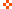Icon Legend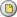New Messages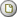No New Messages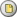Hot Topic w/ New Messages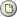Hot Topic w/o New Messages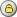Locked w/ New Messages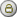Locked w/o New Messages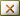Post New ThreadReply to MessagePost New PollSubmit VoteDelete My Own PostDelete My Own ThreadRate Posts